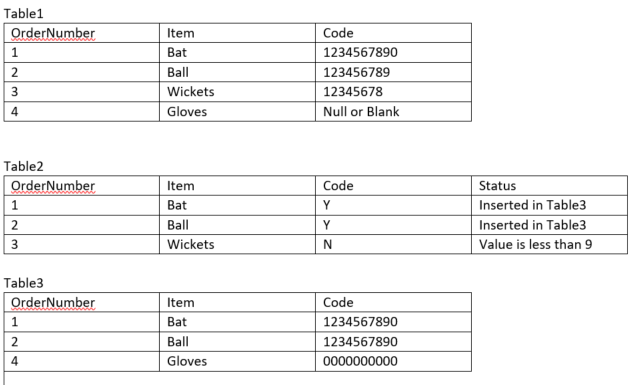# Insert Update QueryI have two tables Table1 and Table 2,
I want to insert data into Table 3 from Table 1
and update Table 2

Insert into Table3(OrderNumber, Item, Code)
Select
OrderNumber,
Item,

If the item is Code is null or zero in Table 1
Insert table 3 Code with 0000000000

If the length of the code is 10 in table 1 then insert in table 3
If the length of the code is 9 in table 1 then append 0 to it and then insert In table 3
If the length of the code is less than 9, then UPDATE table2 code and status to N/ Value is less than 9

From
Table 1